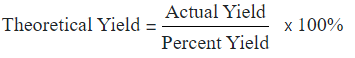Request a Tool

Theoretical Yield Calculator

The amount of product resulting from a seamless but theoretical chemical reaction

Output

Theoretical Yield
0

FormulaDefination / Uses

The theoretical yield is the maximum amount of product that can be generated from a given amount of reactants. When calculating the theoretical yield of a chemical reaction, this is a technique to use.

The quantity of a products formed from full conversion of the limiting reactant in a chemical process is known as theoretical yield. It's the quantity of product produced by a continuous but hypothetical chemical process.
As a consequence, theoretical yield differs from the amount we would essentially obtain from a laboratory reaction. It is most commonly expressed in moles or grammes.Use the upper given formula for manual calculations. No sign-up, registration OR captcha is required to use this tool.